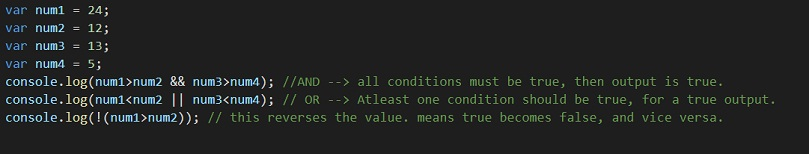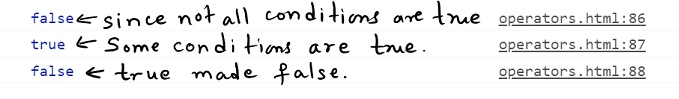# Logical Operators in Javascript

Javascript Logical operators

We are going to use these operators for finding logic between values. Simply speaking, we are going to perform logical operations here. It is very simple, and useful too. As we move forward and have some programs, we will understand the broad idea, and get familiar with the concept.

## Logical Operators in Javascript

Well, sometimes, when we need to compare multiple things together, we are going to make use of different logical operators. Let’s say that it is raining outside, AND you want to go outside urgently. Now, if both cases are true, then only you are going to wear a raincoat. So, in short, we are checking multiple conditions, so that we can do something.

From the above example, we can understand that we are deciding whether or not to wear a raincoat, based on multiple things like whether is it raining outside, and whether we also want to go outside. Notice that if all the conditions are true, then only, the resultant is true, and then only, we are going to do something.

Here are some Logical operators in Javascript, that we are going to have a look on-

 Operator Description && This is logical AND operator. This returns true only if all the values or comparisons made are true. || This is the logical OR operator. This returns true if at least one value in comparison is true. ! This is the logical NOT operator. It converts true to false and false to true. So now we understand that! true is false.

The following program is for understanding the Logical operators in Javascript.You can simply try the above program. All we are doing is that first, we are trying the AND logic like both the values should be true for a true output. Next, we are trying the OR thing, which says that any one of the given comparisons should be true, for a true output, and the NOT thing, which reverses the output, like if it is true, we give it as false.

Have a look at the output of the given program →The above example is pretty standard, and we are going to understand briefly, what is happening.

In the first case, we are checking two things. First, num1 is greater than num2 AND num3 is greater than num4. Now, this is going to return true, if and only if both the conditions are true. This makes sense as well when we say AND!

Next, we are checking for other two things. Here, num1 is less than num2, OR num3 is less than num4. This is going to return true if either of the things returns true, which means any one of the comparisons should be true.

Lastly, we are making use of the NOT operator. Through this, we are going to reverse the output. This means that if the output was true, then NOT true is false, or NOT false is true. In the above comparison, num1> num2 is true, so NOT true is false.